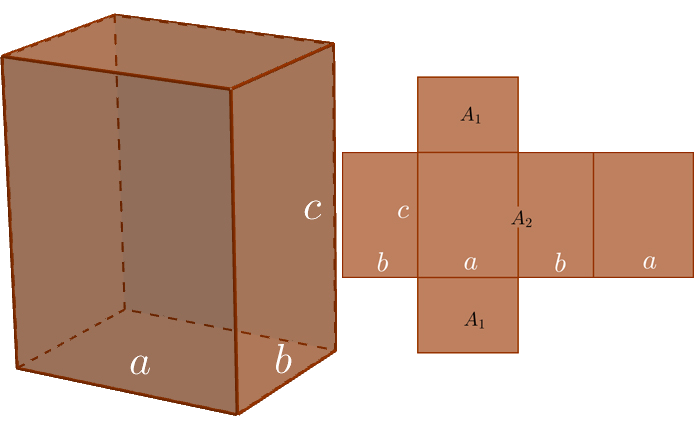# PrismArea

$A=2{A}_{1}+{A}_{2}=2ab+2c\left(a+b\right)$

Base Area

${A}_{1}=ab$

Side Area

${A}_{2}=2c\left(a+b\right)$

Volume

$V={A}_{1}·c=abc$
Keywords: prism, area, volume